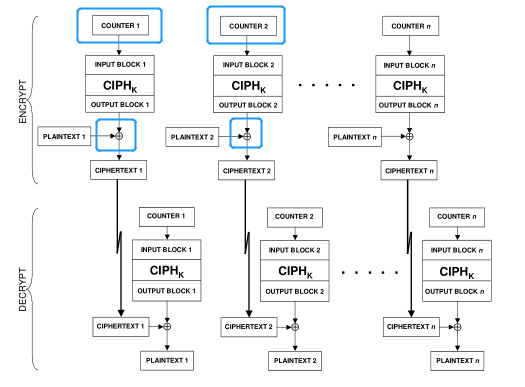# 内联汇编的妙用

## 一、问题描述

AES加密模式中，有一种CTR模式，其流程如下图：int NICE_aesEncrypt_ctr(const uint8_t *key, uint32_t keyLen, const uint8_t *vi,
const uint8_t *pt, uint8_t *ct, uint32_t len)
{
//拷贝初始向量
uint8_t counter={0};
memcpy(counter, vi, keyLen);

uint8_t *pos = ct;
for(int i=0; i<len; i+=BLOCKSIZE){
NICE_encrypt();                 //加密
NICE_xorvi((int)pt);            //明文异或
NICE_storestate((int)pos);      //保存密文
pos +=BLOCKSIZE;                //下一个密文位置
pt  +=BLOCKSIZE;                //下一个明文位置
counter+= 1;                //**计数器加1**
}
return 0;
}


## 三、Debug

### 2、利用数据相关性（失败）

// 5 ctr
int NICE_aesEncrypt_ctr(const uint8_t *key, uint32_t keyLen, const uint8_t *vi, const uint8_t *pt, uint8_t *ct, uint32_t len) {
uint8_t *counter =(char*)malloc(sizeof(char)*16);
memcpy(counter, vi, keyLen);
uint8_t temp =counter;  //**增加临时变量**
uint8_t *pos = ct;

int zero=0;
for(int i=0; i<len; i+=BLOCKSIZE){
temp += i%16;       //**与循环变量有关**
NICE_encrypt();
NICE_xorvi((int)pt);
NICE_storestate((int)pos);
pos +=BLOCKSIZE;
pt  +=BLOCKSIZE;
//counter+=1;
}
return 0;
}


### 3、内联汇编（结果正确）

// 5 ctr
int NICE_aesEncrypt_ctr(const uint8_t *key, uint32_t keyLen, const uint8_t *vi, const uint8_t *pt, uint8_t *ct, uint32_t len) {
uint8_t *counter =(char*)malloc(sizeof(char)*16);
memcpy(counter, vi, keyLen);
uint8_t *pos = ct;

int zero=0;
counter-=1;  //**预先处理**
for(int i=0; i<len; i+=BLOCKSIZE){
//前面三条完成+1操作，后面一条完成加密
__asm__ __volatile__(
"  lb x10,15(%[src1]) \n\t"
"  sb x10,15(%[src1]) \n\t"
"  .insn r 0x7b,2,0x12,x0,%[src1],x0 \n\t"
:"=r"(zero)
:[src1]"r"(counter)
);
NICE_encrypt();
NICE_xorvi((int)pt);
NICE_storestate((int)pos);
pos +=BLOCKSIZE;
pt  +=BLOCKSIZE;
}
return 0;
}